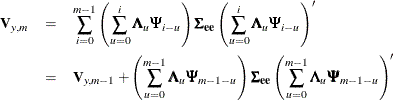The STATESPACE Procedure

Forecasting

Given estimates of
,, and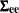, forecasts of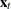are computed from the conditional expectation of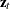.

In forecasting, the parameters F, G, andare replaced with the estimates or by values specified in the RESTRICT statement. One-step-ahead forecasting is performed for the observation, where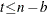. Hereis the number of observations and b is the value of the BACK= option. For the observation, where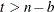, m-step-ahead forecasting is performed for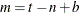. The forecasts are generated recursively with the initial condition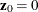.

The m-step-ahead forecast of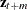is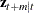, wheredenotes the conditional expectation ofgiven the information available at time t. The m-step-ahead forecast of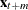is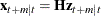, where the matrix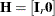.

Let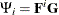. Note that the lastelements ofconsist of the elements of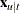for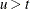.

The state vectorcan be represented as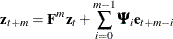Since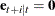for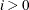, the m-step-ahead forecastis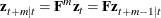Therefore, the m-step-ahead forecast ofis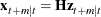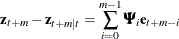The variance of the m-step-ahead forecast error is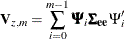Letting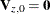, the variance of the m-step-ahead forecast error of,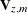, can be computed recursively as follows: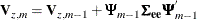The variance of the m-step-ahead forecast error ofis theleft upper submatrix of; that is,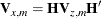Unless the NOCENTER option is specified, the sample mean vector is added to the forecast. When differencing is specified, the forecasts x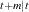plus the sample mean vector are integrated back to produce forecasts for the original series.

Let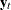be the original series specified by the VAR statement, with some 0 values appended that correspond to the unobserved past observations. Let B be the backshift operator, and let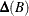be thematrix polynomial in the backshift operator that corresponds to the differencing specified by the VAR statement. The off-diagonal elements of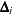are 0. Note that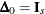, where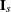is theidentity matrix. Then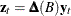.

This gives the relationship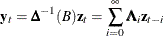where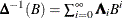and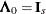.

The m-step-ahead forecast of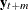is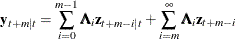The m-step-ahead forecast error ofis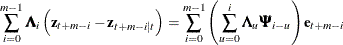Letting, the variance of the m-step-ahead forecast error of,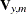, is